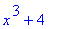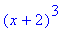# Math 1050 - MAPLE LAB II

Maple Lab II

## Math 1050 - MAPLE LAB 2

 Math 1050 - Precalculus - New Graphs from Old

Translations:
Vertical - The function f(x) is vertically translated through the operation f(x)+c or f(x)-c for c>0. The operation f(x)+c slides the original graph up c units, while f(x)-c slides the graph down c units. For example look at the function f(x)=x3. How will this compare to f(x)+4=x3+4  and f(x)-2=x3-2? To see the changes we can graph all three on the same axis. Start by defining the function f(x) in MAPLE.

> f:=x->x^3;> plot({f(x),f(x)+4,f(x)-2},x=-3..3,y=-10..10,color=[blue,red,tan]);The top line represents the function f(x)+4, the middle line is the original function f(x)=x3, and the bottom line is the function f(x)-2. Notice they all have the same shape, they are just shifted up and down the y axis by c units. Notice that MAPLE was directed to use the colors blue, red and tan in the graph, this was done only because the default colors include yellow when there are more than two equations per graph, and the yellow line is difficult to see on the printout. However, be careful, the first function graphed is not the blue one.

If you would like to see the algebraic representation of your functions, you can find them using MAPLE, and then simplify if necessary. To have MAPLE find the algebraic representation of f(x)+4 the command would be as follows.

> f(x)+4;Horizontal - The function f(x) is horizontally translated through the operation f(x+c) or f(x-c) for c>0. However, the operation f(x+c) slides the graph c units to the left along the x axis, while f(x-c) slides the graph c units to the right. Let's look at the same function f(x)= x3 and compare it to f(x+2)=(x+2)3  and f(x-4)=(x-4)3.

> plot({f(x),f(x+2),f(x-4)},x=-6..6,y=-10..10,color=[blue,red,tan]);The graph on the left represents the function f(x+2). The original graph of f(x) (found in the middle) has been moved 2 units to the left. The graph on the right represents f(x-4), the original graph has been moved 4 units to the right. So f(x+c) moves the graph back c units in the x direction. Again to see the algebraic representation of f(x+2) use MAPLE.

> f(x+2);If we had wanted Maple to multiply this out for us, we would use the expand command.

Stretching and Shrinking:
Vertical - The function g(x) is vertically stretched or shrunk through when g(x) is multiplied by a . If a>1 the graph of a*g(x) will be stretched away from the x axis by a factor of a, while if 02+4 to see how 3g(x)=3(-x2+4) and 1/2g(x)=1/2(-x2+4) will compare to the original. First define the function g(x) in MAPLE, then plot the three functions on the same axis.